# GRE Subject Test: Math : Chain Rule

## Example Questions

### Example Question #11 : Derivatives & Integrals

Compute the derivative: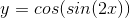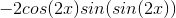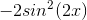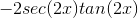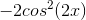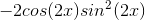Explanation:

This question requires application of multiple chain rules.  There are 2 inner functions in, which are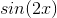and.

The brackets are to identify the functions within the function where the chain rule must be applied.

Solve the derivative.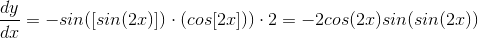The sine of sine of an angle cannot be combined to be sine squared.### Example Question #2 : Chain Rule

Find the derivative of the following function: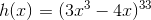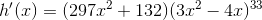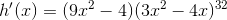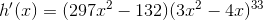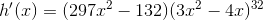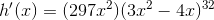Explanation:

Recall chain rule for this problem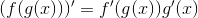So if we are given the following,We can think of it like this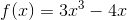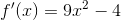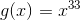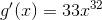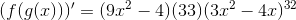Clean it up a bit to get: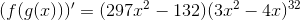### Example Question #1 : Chain Rule

What is the derivative of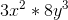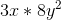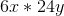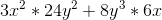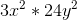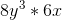Explanation:

Chain Rule: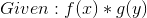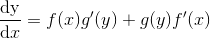For this problem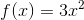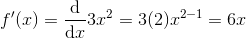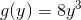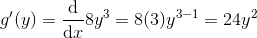Plug the values into the Chain Rule formula and simplify: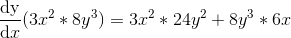### All GRE Subject Test: Math Resources